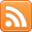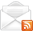# ASP.NET,C#.NET,VB.NET,JQuery,JavaScript,Gridview

aspdotnet-suresh offers C#.net articles and tutorials,csharp dot net,asp.net articles and tutorials,VB.NET Articles,Gridview articles,code examples of asp.net 2.0 /3.5,AJAX,SQL Server Articles,examples of .net technologies

### jQuery Difference between == and === in JavaScript with Example

Jan 11, 2016
Introduction

Here I will explain difference between == and === in
JavaScript with example or what is the difference between == and === in jQuery with example. Generally JavaScript will provide different type of operators those are strict equality (===) and type converting equality (==). These operators help us to understand difference between == and === in JavaScript or jQuery.

Description: jQuery hide div if we click outside of it example and many articles relating to css, AngularJS, . Now I will explain difference between == and === in or jQuery with example.

Generally JavaScript will provide different type of operators those are strict equality and type converting equality.

Strict equality (===) means values which we are comparing must have same type that means "2" not equal to 2 ("2"===2 it will return false).

Type converting equality (==) means automatically it will covert variable to value either it's string or number that means "2" is equal to 2 ("2" == 2 it will return true)

Here double equal (==) is an auto type converting equality and three equal (===) is strict equality operator that means it will not covert values automatically.

Here we will learn with simple examples

Example1

 1=="1" // it will return true because here string will converted as number 1 === "1" // it will return false because here 1 is number and "1" is string
Example2

 0 == false // it will return true because here false is equivalent of 0 0 === false // it will return false because both are different operands
If you want to check it in complete example you need to write the code like as shown below

 difference between == and === in javascript
Live Demo

Check following demo to check difference between == and === in jQuery

 If you enjoyed this post, please support the blog below. It's FREE! Get the latest Asp.net, C#.net, VB.NET, jQuery, Plugins & Code Snippets for FREE by subscribing to our Facebook, Twitter, RSS feed, or by email.Subscribe by RSSSubscribe by EmailPrajesh Dasari said...

Very simple and good one. Thank you.

sarath said...

Good one..

Unknown said...

Good one.